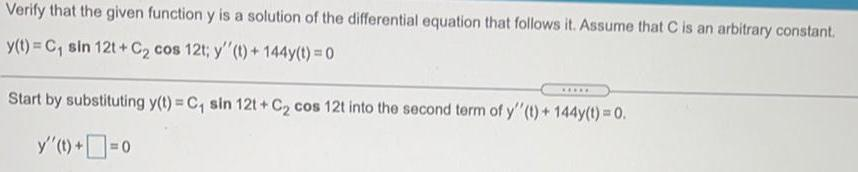Question:

# Verify that the given function y is a solution of theVerify that the given function y is a solution of the differential equation that follows it. Assume that C is an arbitrary constant. y(t) = C₁ sin 12t+C₂ cos 12t; y''(t) + 144y(t) = 0 ***** Start by substituting y(t) = C₁ sin 12t+C₂ cos 12t into the second term of y''(t) + 144y(t) = 0. y' (t) +=o